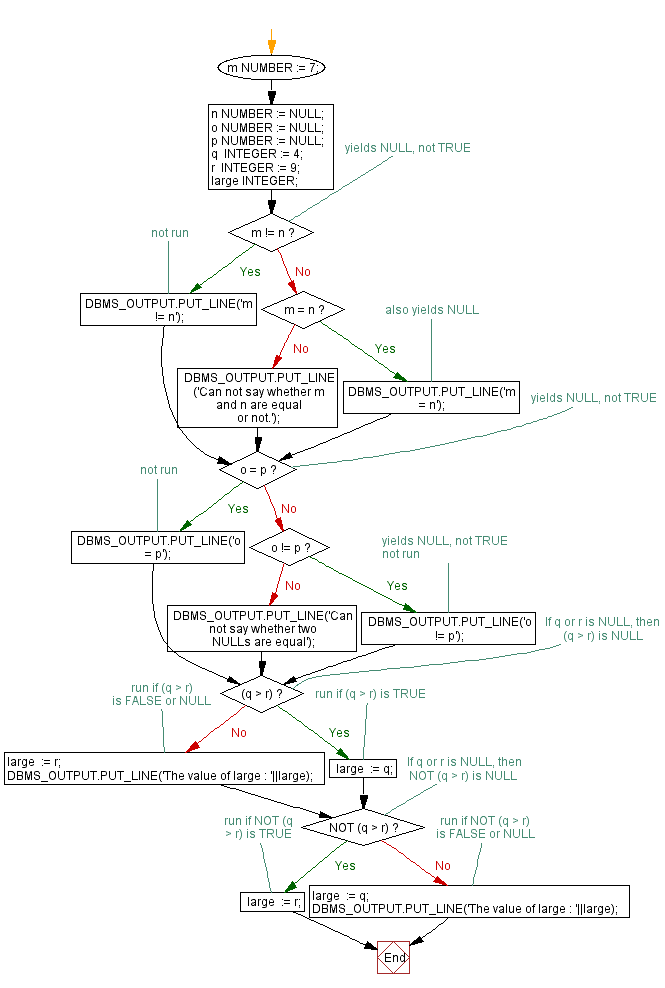﻿ PL/SQL Fundamentals Exercises: PL/SQL block to describe NULL values in unequal comparison - w3resource# PL/SQL Fundamentals Exercises: PL/SQL block to describe NULL values in unequal comparison

## PL/SQL Fundamentals: Exercise-15 with Solution

Write a PL/SQL block to describe the usage of NULL values in equal comparison, unequal comparison and NOT  NULL equals NULL comparison.

In the following example the m and n seem unequal. But, NULL values are indeterminate. Whether m equals n is unknown. Therefore, the IF condition yields NULL and the sequence of statements is bypassed, similarly o and p seem equal. But, again, that is unknown, so the IF condition yields NULL and the sequence of statements is bypassed and however, if either q or r is NULL, then the first IF statement assigns the value of r to large and the second IF statement assigns the value of q to large.

PL/SQL Code:

``````DECLARE
m NUMBER := 7;
n NUMBER := NULL;

o NUMBER := NULL;
p NUMBER := NULL;

q    INTEGER := 4;
r    INTEGER := 9;

large INTEGER;

----------------------------------
BEGIN
IF m != n THEN  -- yields NULL, not TRUE
DBMS_OUTPUT.PUT_LINE('m != n');  -- not run
ELSIF m = n THEN -- also yields NULL
DBMS_OUTPUT.PUT_LINE('m = n');
ELSE
DBMS_OUTPUT.PUT_LINE
('Can not say whether m and n are equal or not.');
END IF;

-----------------------------------
IF o = p THEN  -- yields NULL, not TRUE
DBMS_OUTPUT.PUT_LINE('o = p');  -- not run
ELSIF o != p THEN  -- yields NULL, not TRUE
DBMS_OUTPUT.PUT_LINE('o != p');  -- not run
ELSE
DBMS_OUTPUT.PUT_LINE('Can not say whether two NULLs are equal');
END IF;
--------------------------------------
IF (q > r)       -- If q or r is NULL, then (q > r) is NULL
THEN large  := q;  -- run if (q > r) is TRUE
ELSE large  := r;  -- run if (q > r) is FALSE or NULL
DBMS_OUTPUT.PUT_LINE('The value of large : '||large);
END IF;

IF NOT (q > r)   -- If q or r is NULL, then NOT (q > r) is NULL
THEN large  := r;  -- run if NOT (q > r) is TRUE
ELSE large  := q;  -- run if NOT (q > r) is FALSE or NULL
DBMS_OUTPUT.PUT_LINE('The value of large : '||large);
END IF;

END;
/
```
```

Sample Output:

```Can not say whether m and n are equal or not.
Can not say whether two NULLs are equal
The value of large : 9

Statement processed.

0.00 seconds
```

Flowchart:Improve this sample solution and post your code through Disqus

What is the difficulty level of this exercise?

﻿